North East population changes

In 2020, the total population of North East region was 2.4M and it increased by 140k people since 2002. That's 6.2% population growth . Population average age was 41.6 and it increased by 2.2 years since 2002. The region was ageing faster than England and Wales in which the age grew by 1.7 years in the same period. The population was growing slower than population in England and Wales which grew by 13.5%.

+140k

population growth since 2002

+6.2%

population growth since 2002

+2.2 yrs

average age change
since 2002

Compares speed of population growth to other regions. Areas with the highest population growth are on the left. Shows relative population change between 2002 and 2020.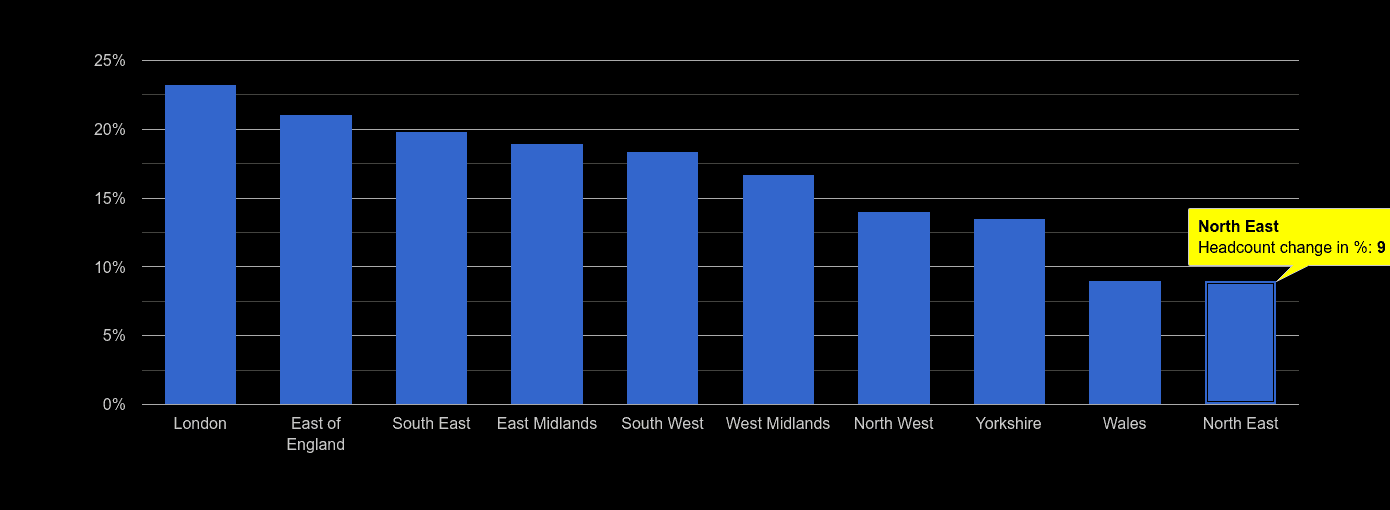Compares the latest population estimates with population estimates in 2002 and shows percentage difference.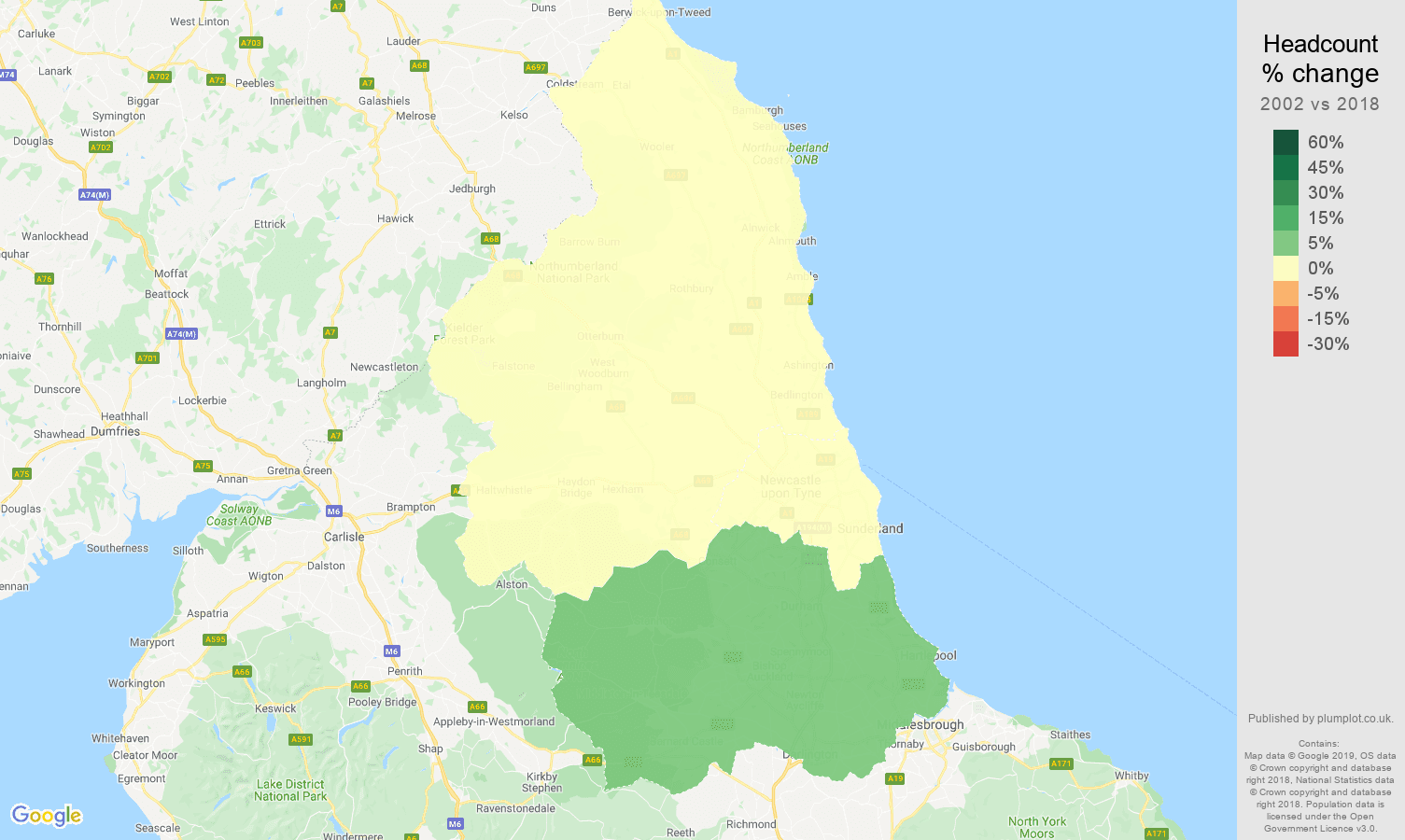North East population growth rate

Shows year over year population change.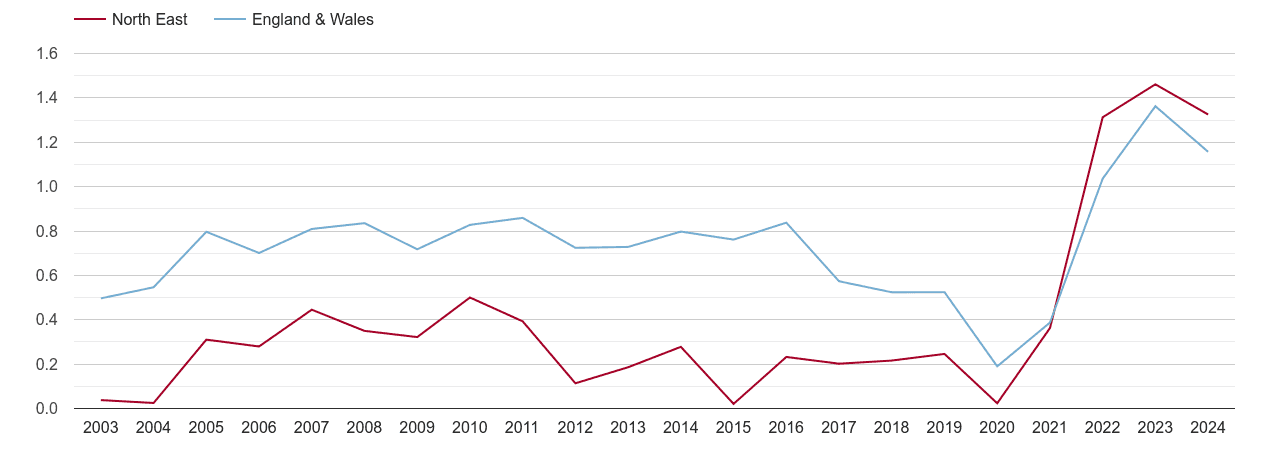North East population growth

Shows total population by year.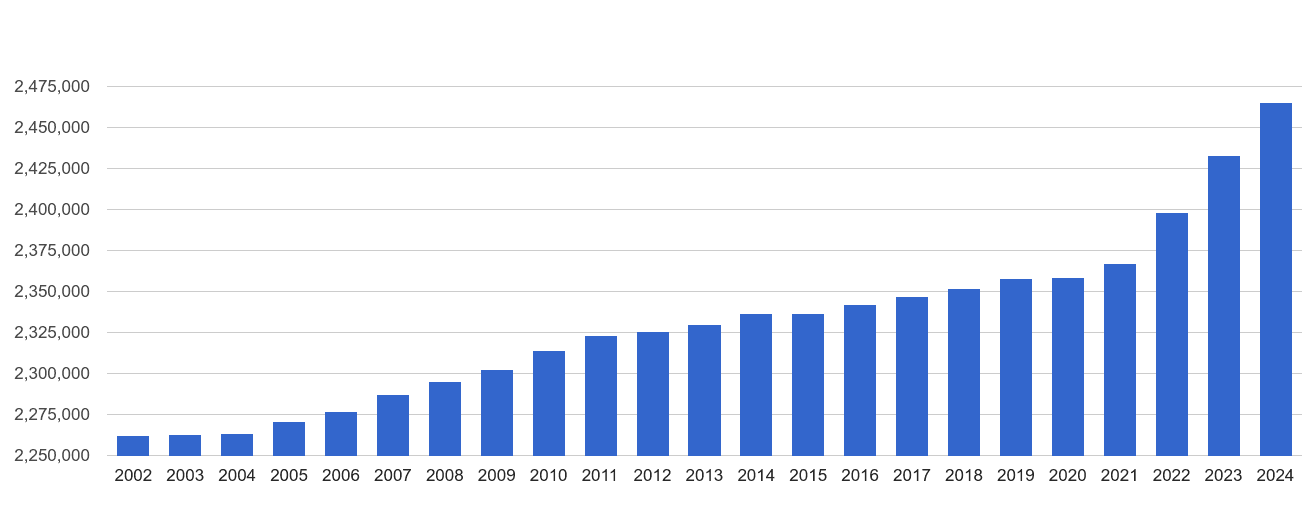North East average age change map

Shows population's average age change since 2002.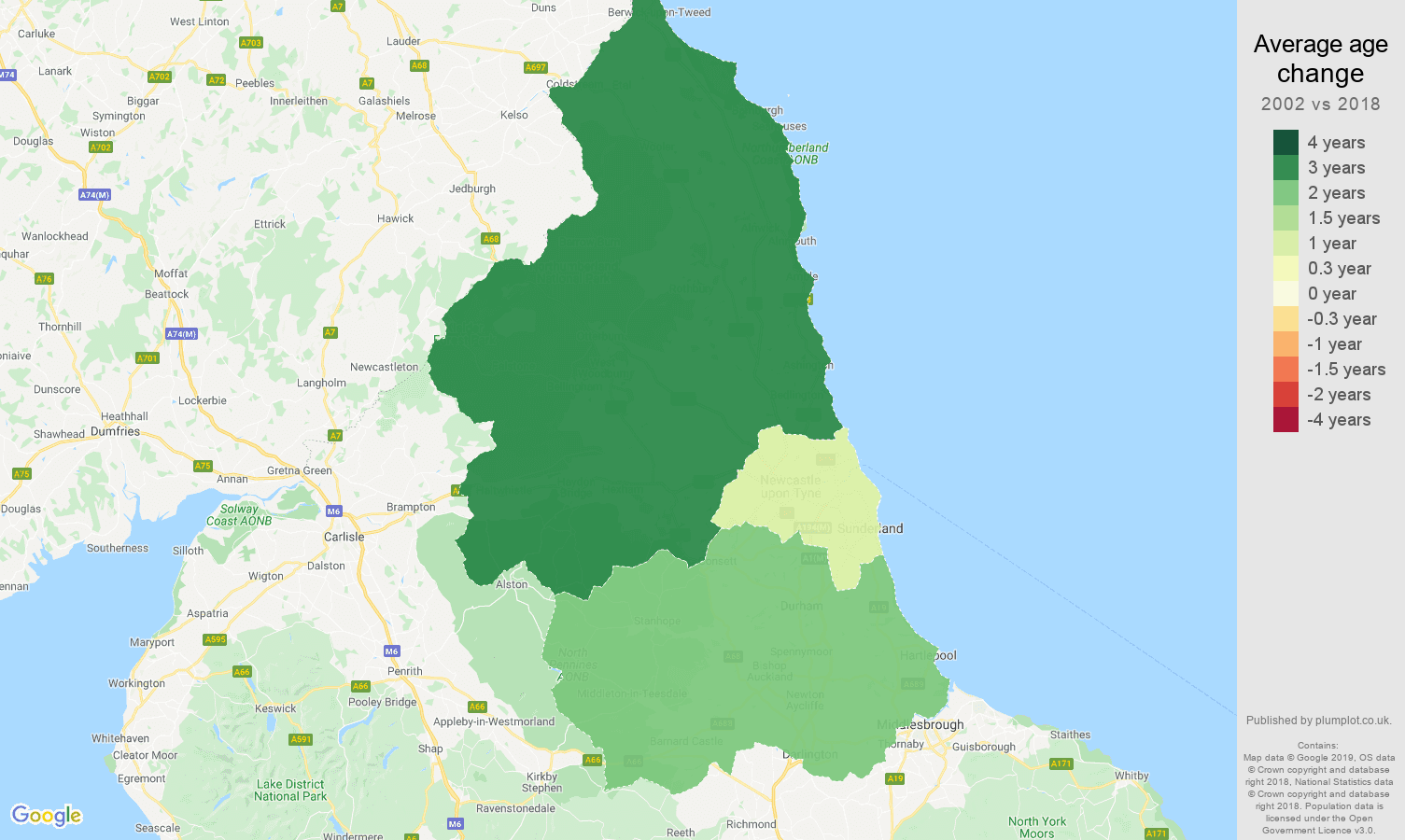North East population average age change rank

Compares the speed of average age change in the regions. Areas with the fastest ageing population are on the left, the areas with their population becoming younger are on the right. Compares change between 2002 and 2020.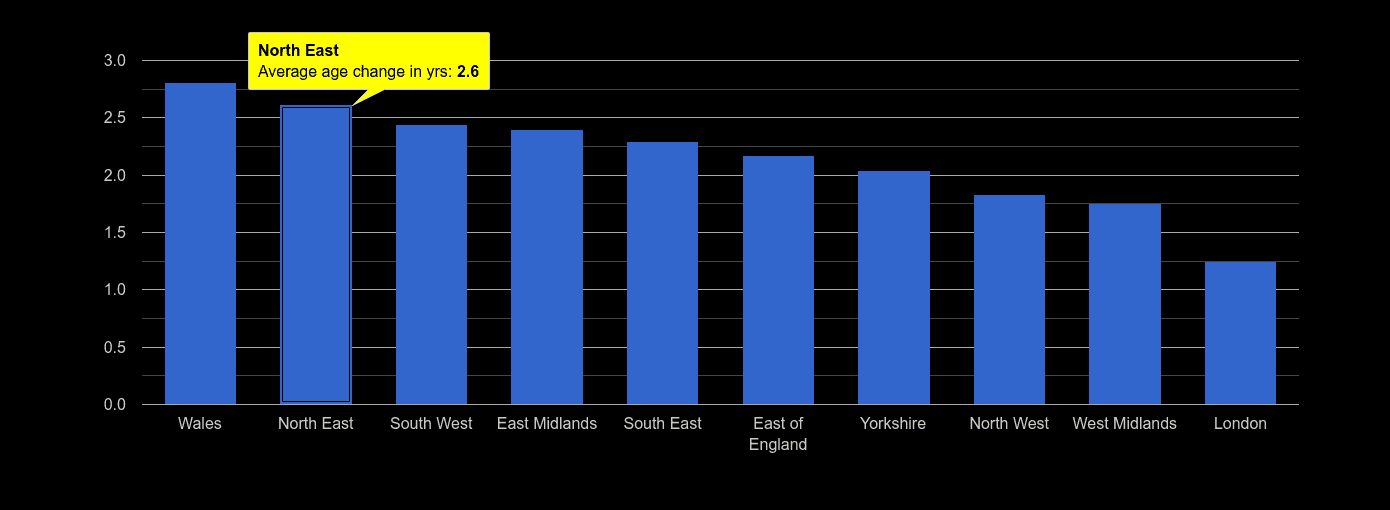Plumplot area insights - interactive charts and mapsNorth East population average age

Annual statistics of population's average age.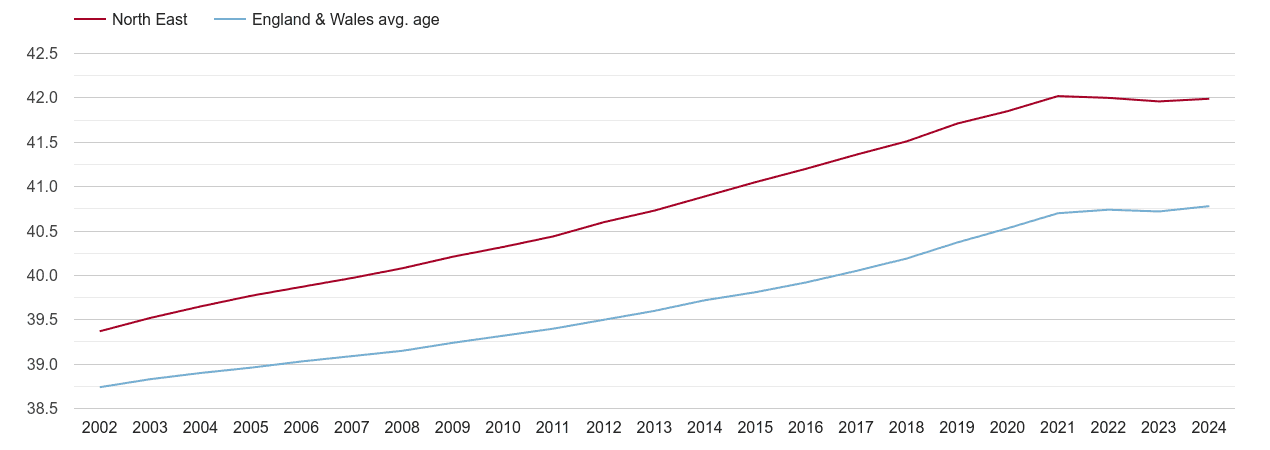North East male and female average age

Annual statistics of population's average age by sex.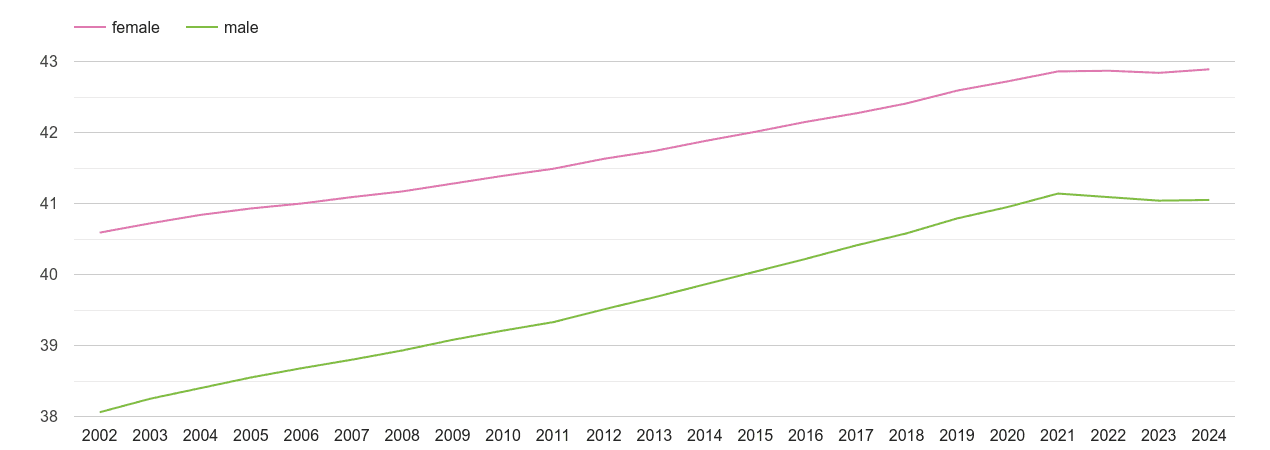North East population changes compared to other regions

Comparison to other regions. Metrics show a percentage change in the headcount between 2002 and 2020 on y-axis and change of the population's average age on x-axis. The size of the circle is directly proportional to the total population in 2020.

Population average age change